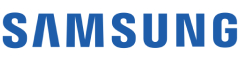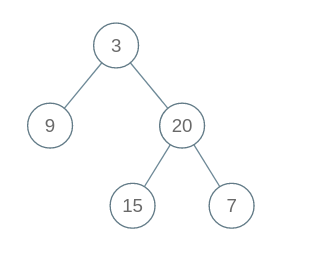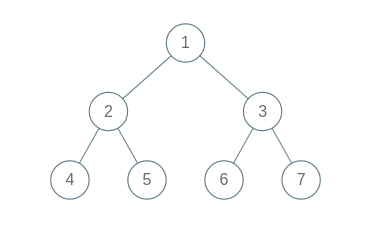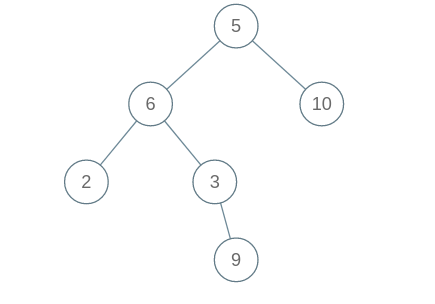New update is available. Click here to update.
Topics

# Construct Binary Tree from Inorder and Postorder Traversal

Moderate0/80
Average time to solve is 25m## Problem statement

You are given arrays 'inOrder' and 'postOrder', which represent 'inorder' traversal and 'postorder' traversal of a 'Binary Tree' respectively.

Construct a 'Binary Tree' represented by the given arrays and return it's head.

Note:
``````Assume that the Binary Tree contains only unique elements.
``````

Example:
``````Input: 'inOrder' = [9, 3, 15, 20, 7], 'postOrder' = [9, 15, 7, 20, 3]

Output:
We get the following binary tree from Inorder and Postorder traversal:
``````Detailed explanation ( Input/output format, Notes, Images )
Sample Input 1:
``````7
4 5 2 6 7 3 1
4 2 5 1 6 3 7
``````

Output on console:
``````1 2 3 4 5 6 7
``````

#### Explanation for Sample Output 1:

``````We get the following Binary Tree from the given Inorder and Postorder traversal:
``````Sample Input 2:
``````6
2 9 3 6 10 5
2 6 3 9 5 10
``````

Sample Output 2:
``````5 6 10 2 3 9
``````

#### Explanation for Sample Output 2:

``````We get the following Binary Tree from the given Inorder and Postorder traversal:
``````Expected Time Complexity:
``````Try to solve this in O(n).
``````

Constraints :
``````1 <= 'n' <= 10000
1 <=  'inOrder[i]' , ‘postOrder[i]’ <= 100000

Time Limit: 1 second
``````Console